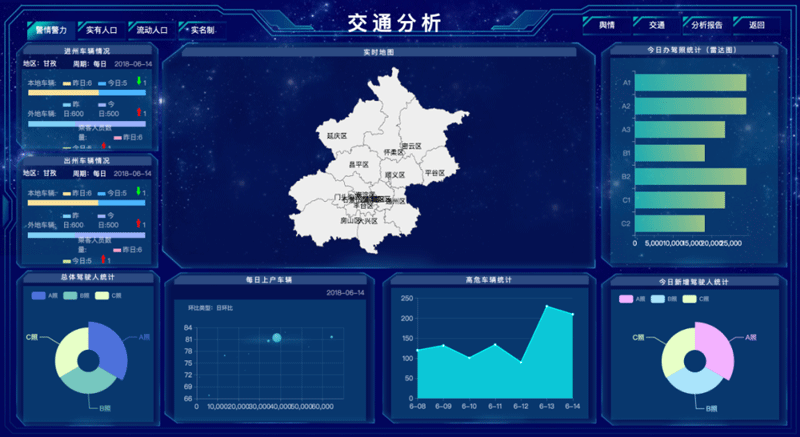﻿ 利用python实现可视化大屏_python_脚本之家
python# 利用python实现可视化大屏## 1、实践之轨迹地图

```import  os
import plotly.graph_objects as go
import plotly as py

mapbox_access_key = '需要你自己去mapbox的网站去申请一个账号'
style = 'streets'
# 采用的风格为streets类型

fig = go.Figure()
color_map = ['#7bd3f6', '#bcbd22', '#17becf','#d62728']
lat_li = []
lng_li = []
for item in [(122.180204,30.806457),(123.256865,29.762908),(123.366728,28.631261),(122.048368,26.350981),(120.048857,24.705378),(119.060087,22.835386),(119.543486,19.433918),(119.960966,16.276336),(116.159697,11.413759),(110.183134,5.067372),(105.173368,0.064226),(101.833525,1.909575),(101.833525,1.909575),(101.833525,1.909575),(88.122587,3.840643),(80.300322,5.154913),(76.433134,7.338996),(71.247587,13.900433),(63.425322,22.246862),(56.394072,25.857701)]:
lng_li.append(item)
lat_li.append(item)

name='vessel',
mode='markers+lines',
lon=lng_li,
lat=lat_li,
marker={'size': 2, 'color': color_map},
showlegend=False
))

fig.update_layout(
margin={'l': 0, 't': 0, 'b': 0, 'r': 0}
, mapbox={
'center': {'lon': 90, 'lat': 8},
'zoom': 1
, 'style': style
, 'accesstoken': mapbox_access_key}
)

if os.path.isfile(url):
os.remove(url)
py.offline.plot(fig, filename=url, auto_open=False)
# 保存为html文件```## 2、最终制作出来的效果图## 3、大屏的由来